One of the most popular posts on this site is from a couple of years ago, about using expectation-maximization (EM) to estimate the parameters for data sampled from a mixture of Gaussians. In this post I will revisit Gaussian Mixture Modeling (GMM) using Pyro, a probabilistic programming language developed by Uber AI Labs.1

The Pyro documentation contains a GMM tutorial that I extend here by making the data generating process two-dimensional (as in the EM post mentioned above).2 The samples are generated from two Gaussian distributions with the same parameters as before:

Here is what these samples look like, colored by the cluster from which they originated: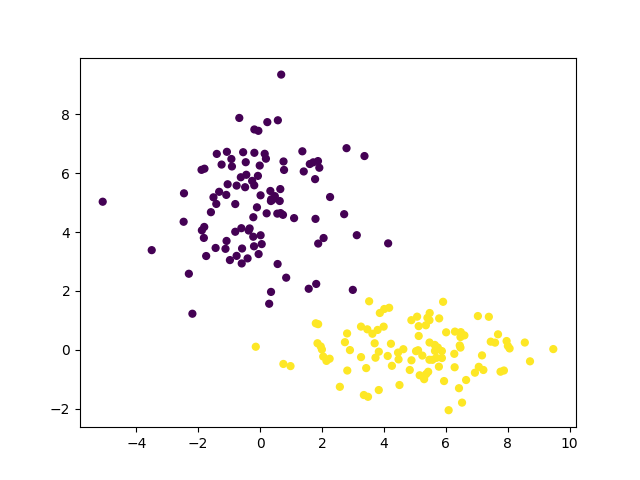The model that we set up in Pyro consists of three parameters: weights (the proportion of samples that originate from each Gaussian), locations (means of the normal distributions) and scales (the covariance matrix for each Gaussian):

There are two interesting things about this model: first, we supplied it with intentionally bad guesses of the parameters to estimate. Second, we did not supply the model with useful priors about these parameters (e.g. Gamma-distributed covariance matrix entries). Pyro readily supports modeling with Bayesian priors, but they are not necessary in this case.

In addition to the parameters listed above, we also model the assignment of each data point to one of the two Gaussian distributions in our mixture. In our model the assignment is used as follows:

The corresponding portion of the guide (which is used to approximate all unobserved sampling distributions in the model) uses an intermediate variable called assignment_probs:

With this setup, we are ready to use variational inference to estimate our model parameters. The following plots (created at the first iteration and every 50th iteration thereafter) illustrate how the model converges within 150 iterations: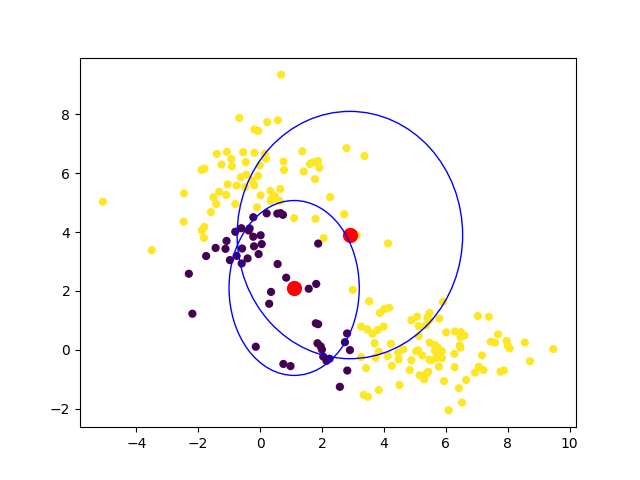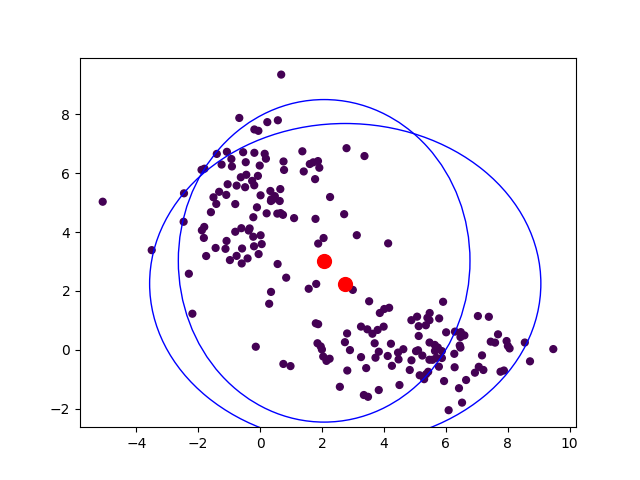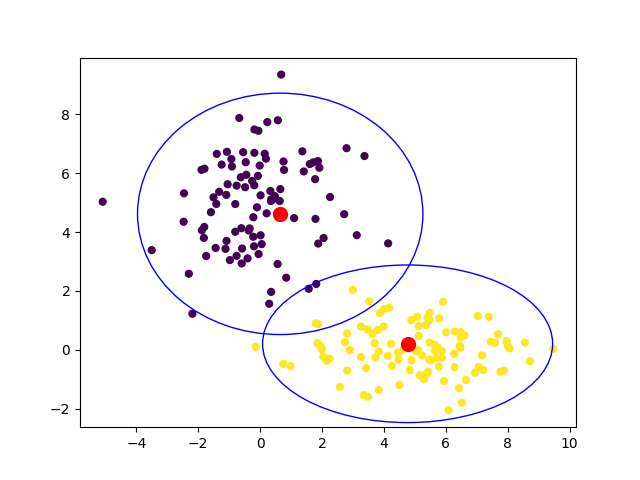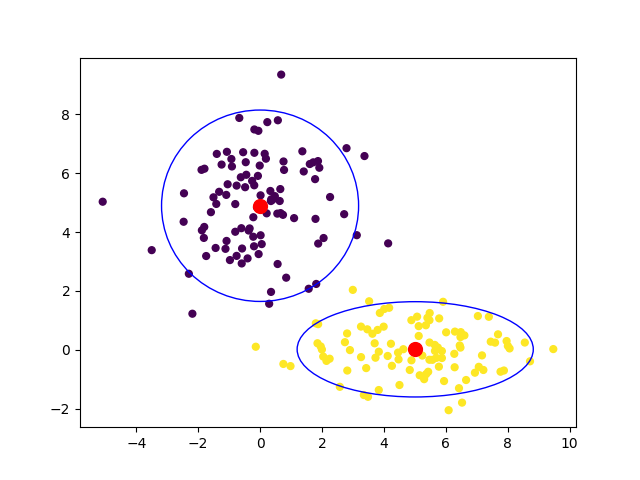The final parameter estimates (including the cluster assignments as indicated in the plots above) are fairly accurate given the relatively small amount of data, short running time, and intentionally poor initialization:

locs: [[-0.0007,  4.8963],
[ 5.0099,  0.0136]]
scales: [[[2.5315, 0.0000],
[0.0000, 2.6476]],

[[3.6354, 0.0000],
[0.0000, 0.6563]]]
weights = [0.4976]


This example shows how easy it is to get started with Pyro, and gives a sense of how powerful probabilistic programming is for this type of modeling. For more about clustering with EM and other algorithms, see this talk.

1. As always, the content of this site is a result of work done in my personal time and does not necessarily reflect the views of Uber or any other party. I am acquainted with the developers of Pyro, but this post was written solely out of personal interest without the use of Uber resources. Eli and I gave a presentation together at Duke a few years ago about the Indian buffet process described here and Fritz and JP have visited our Boulder office to help apply Pyro to mapping-related problems.